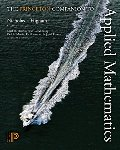libib

Applied MathematicsThis is the most authoritative and accessible single-volume reference book on applied mathematics. Featuring numerous entries by leading experts and organized thematically, it introduces readers to applied mathematics and its uses; explains key concepts; describes important equations, laws, and functions; looks at exciting areas of research; covers modeling and simulation; explores areas of application; and more. Modeled on the popular Princeton Companion to Mathematics, this volume is an indispensable resource for undergraduate and graduate students, researchers, and practitioners in other disciplines seeking a user-friendly reference book on applied mathematics. Features nearly 200 entries organized thematically and written by an international team of distinguished contributors Presents the major ideas and branches of applied mathematics in a clear and accessible way Explains important mathematical concepts, methods, equations, and applications Introduces the language of applied mathematics and the goals of applied mathematical research Gives a wide range of examples of mathematical modeling Covers continuum mechanics, dynamical systems, numerical analysis, discrete and combinatorial mathematics, mathematical physics, and much more Explores the connections between applied mathematics and other disciplines Includes suggestions for further reading, cross-references, and a comprehensive index

No reviews
Item Posts
No posts